#### 期刊菜单

The Research of Dense decoy Target Generation and Feature Vector Extraction
DOI: 10.12677/JISP.2022.112008, PDF, HTML, XML, 下载: 42  浏览: 74

Abstract: Dense decoy target is a new type of active decoy jamming, which has been widely used in military field due to its excellent jamming effect. Based on this, the detection and recognition of dense false targets has quickly become the focus of research in the field of radar electronic warfare. In this paper, according to the generation mechanism of dense false targets, the working principle and advantages and disadvantages of DRFM are described, and then a method of combining antenna pattern function with radar equation to obtain target and jamming echo model is proposed. According to the actual situation, multiple groups of targets are set to obtain normalized and differential channel echo signals of each target.

1. 引言

2. DRFM工作原理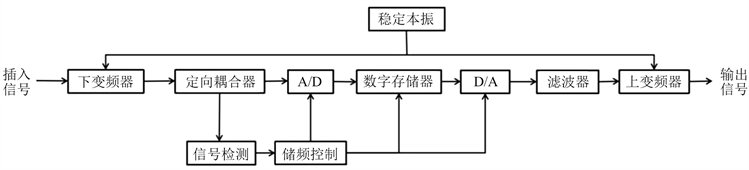Figure 1. The structural representation of DRFM

1) 最小延迟时间短，大约为10 ns~20 ns；

2) DRFM存储的信号不会随着延迟时间的变化而失真，保真度较高；

3) DRFM根据指令可存储和再现多个同时到达的信号，能存储和复制脉冲压缩信号和脉内相位调制的编码信号；

4) 对基准振荡器进行简单的频率偏移，就可以将多普勒频移加入到复制的信号中，从而实现速度欺骗干扰；

5) DRFM既可以在相干干扰机中使用，也可以在某些情况下代替压控振荡器。

DRFM量化信号的方法主要有幅度取样法和相位取样法。其中幅度取样主要包括单通道幅度取样和正交双通道幅度取样，前者结构简单，但对信号的采样频率较高，在带宽较宽时需要时间过长，很难实现，后者在瞬时带宽相同的情况下，只需要前者四分之一的采样频率，但其不足之处在于要求双通道的幅相特性一致以及需要采用正交双通道的上下变频。相位取样将A/D变换器用极性量化器替代，将D/A变换器用加权相加网络替代，在二者的作用下可以完成高频率采样，且具有较大的瞬时带宽，技术实现也较为简单，但是其输出信噪比较低。

3. 目标、干扰回波模型

${S}_{1}=\frac{{P}_{t}{G}_{t}}{4\pi {R}^{2}}$ (1)

${S}_{2}=\frac{{P}_{t}{G}_{t}\sigma }{4\pi {R}^{2}}\cdot \frac{1}{4\pi {R}^{2}}$ (2)

${P}_{r}={A}_{r}{S}_{2}=\frac{{P}_{t}{G}_{t}\sigma {A}_{r}}{{\left(4\pi {R}^{2}\right)}^{2}}$ (3)

$G=\frac{4\pi A}{\lambda }$ (4)

${P}_{r}={A}_{r}{S}_{2}=\frac{{P}_{t}{G}_{t}{G}_{r}{\lambda }^{\text{2}}\sigma }{{\left(4\pi \right)}^{3}{R}^{4}}$ (5)

${P}_{r}={A}_{r}{S}_{2}=\frac{{P}_{t}{G}_{t}{G}_{r}{\lambda }^{\text{2}}\sigma }{{\left(4\pi \right)}^{3}{R}^{4}L}$ (6)

$s\left(t\right)=A\mathrm{exp}\left[j2\pi \left({f}_{c}t+\frac{\mu {t}^{2}}{2}\right)\right]$ (7)

${P}_{t}\left(t\right)={s}^{2}\left(t\right)/{R}_{0}$ (8)

${P}_{r}\left(t\right)={f}^{2}\left(t\right)/{R}_{0}$ (9)

${f}^{2}\left(t\right)=\frac{{s}^{2}\left(t\right){G}_{t}{G}_{r}{\lambda }^{2}\sigma }{{\left(4\pi \right)}^{3}{R}^{4}L}$ (10)

$f\left(t\right)=\sqrt{\frac{{G}_{t}{G}_{r}{\lambda }^{2}\sigma }{{\left(4\pi \right)}^{3}{R}^{4}L}}s\left(t\right)$ (11)

$f\left(t\right)=A\sqrt{\frac{{\lambda }^{2}\sigma }{{\left(4\pi \right)}^{3}{R}^{4}L}}F\left(\theta ,\phi \right)\mathrm{exp}\left[j2\pi \left({f}_{c}t+\frac{\mu {t}^{2}}{2}\right)\right]$ (12)

$f\left(t\right)=\sqrt{\frac{{G}_{t}{G}_{r}{\lambda }^{2}\sigma }{{\left(4\pi \right)}^{3}{R}^{4}L}}s\left(t-{t}_{d}\right)$ (13)

$f\left(t\right)=A\sqrt{\frac{{\lambda }^{2}\sigma }{{\left(4\pi \right)}^{3}{R}^{4}L}}F\left(\theta ,\phi \right)\mathrm{exp}\left[j2\pi \left({f}_{c}\left(t-{t}_{d}\right)+\frac{\mu {\left(t-{t}_{d}\right)}^{2}}{2}\right)\right]$ (14)

${P}_{n}\left(f\right)=\frac{{n}_{0}}{2}\text{\hspace{0.17em}}\text{\hspace{0.17em}}\text{\hspace{0.17em}}\left(-\infty (15)

${P}_{n}\left(f\right)={n}_{0}\text{\hspace{0.17em}}\text{\hspace{0.17em}}\text{\hspace{0.17em}}\left(\text{0} (16)

$R\left(\tau \right)=\frac{{n}_{0}}{2}\delta \left(\tau \right)$ (17)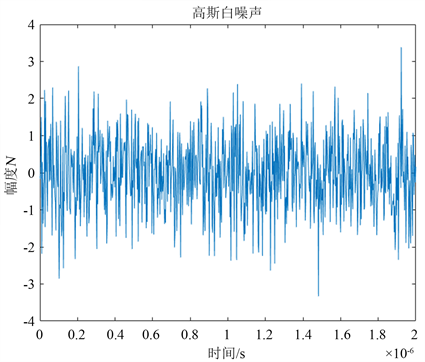Figure 2. The distribution of white Gaussian noise

$f\left(t\right)=A\sqrt{\frac{{\lambda }^{2}\sigma }{{\left(4\pi \right)}^{3}{R}^{4}L}}F\left(\theta ,\phi \right)\mathrm{exp}\left[j2\pi \left({f}_{c}\left(t-{t}_{d}\right)+\frac{\mu {\left(t-{t}_{d}\right)}^{2}}{2}\right)\right]+n\left(t\right)$ (18)

4. 特征向量构建与仿真

$\stackrel{\to }{T}=\left[{\stackrel{\to }{a}}_{1i},{\stackrel{\to }{a}}_{2i},{\stackrel{\to }{a}}_{3i}\right],i=1,2,\cdots ,n$ (19)

$\stackrel{\to }{T}=\left[{\stackrel{\to }{a}}_{1i}/{\stackrel{\to }{a}}_{1i},{\stackrel{\to }{a}}_{2i}/{\stackrel{\to }{a}}_{1i},{\stackrel{\to }{a}}_{3i}/{\stackrel{\to }{a}}_{1i}\right]=\left[1,{\stackrel{\to }{a}}_{2i}/{\stackrel{\to }{a}}_{1i},{\stackrel{\to }{a}}_{3i}/{\stackrel{\to }{a}}_{1i}\right]$ (20)

${f}_{\Sigma }\left(t\right)=A\sqrt{\frac{{\lambda }^{2}\sigma }{{\left(4\pi \right)}^{3}{R}^{4}L}}{F}_{\Sigma }\left(\theta ,\phi \right)\mathrm{exp}\left[j2\pi \left({f}_{c}\left(t-{t}_{d}\right)+\frac{\mu {\left(t-{t}_{d}\right)}^{2}}{2}\right)\right]+n\left(t\right)$ (21)

${f}_{\Delta \theta }\left(t\right)=A\sqrt{\frac{{\lambda }^{2}\sigma }{{\left(4\pi \right)}^{3}{R}^{4}L}}{F}_{\Delta \theta }\left(\theta ,\phi \right)\mathrm{exp}\left[j2\pi \left({f}_{c}\left(t-{t}_{d}\right)+\frac{\mu {\left(t-{t}_{d}\right)}^{2}}{2}\right)\right]+n\left(t\right)$ (22)

${f}_{\Delta \phi }\left(t\right)=A\sqrt{\frac{{\lambda }^{2}\sigma }{{\left(4\pi \right)}^{3}{R}^{4}L}}{F}_{\Delta \varphi }\left(\theta ,\phi \right)\mathrm{exp}\left[j2\pi \left({f}_{c}\left(t-{t}_{d}\right)+\frac{\mu {\left(t-{t}_{d}\right)}^{2}}{2}\right)\right]+n\left(t\right)$ (23)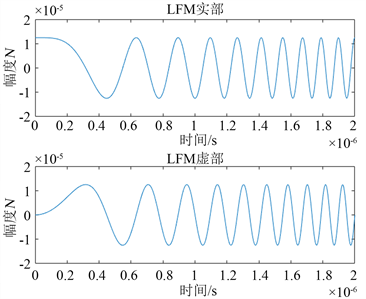Figure 3. The time domain diagram of chirp signal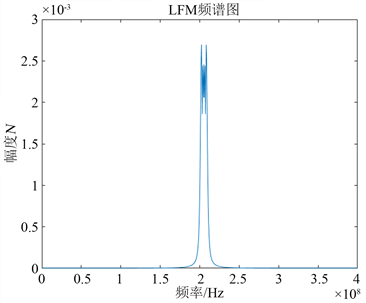Figure 4. The spectrogram of chirp signal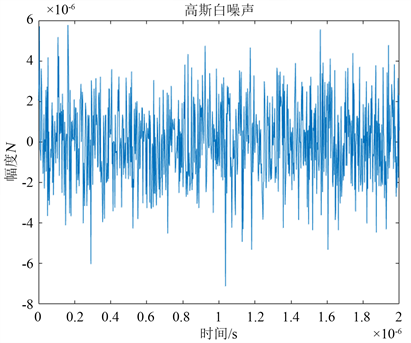Figure 5. Noise audio gram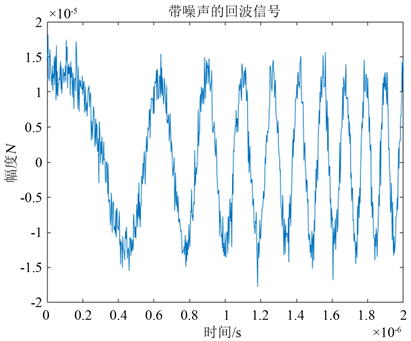Figure 6. The signal waveform with noise

$h\left(t\right)={s}^{\ast }\left(-t\right)$ (24)

$y\left(t\right)={f}_{\Sigma }\left(t\right)\ast h\left(t\right)$ (25)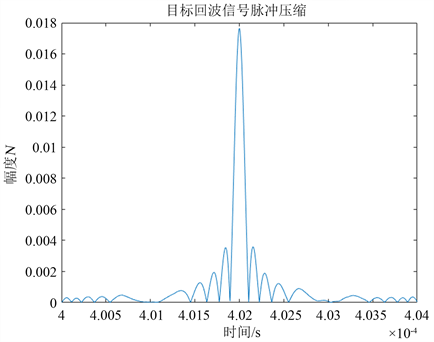Figure 7. The output of ∑channel signal matched filter)Table 1. The incoming direction of objectives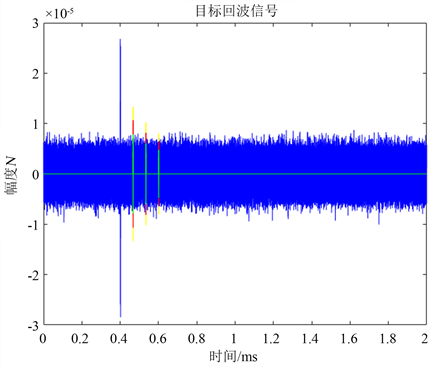Figure 8. The echo signal of ∑channelFigure 9. The matched filtering of ∑channelecho signalTable 2. The time coordinate of extreme point

5. 小结

NOTES

*第一作者。

  李迎春, 王国宏, 孙殿星, 关成斌. 基于主被动雷达数据融合的抗密集假目标干扰技术[J]. 信号处理, 2008(8): 12-17.  周畅, 汤子跃, 朱振波. 基于旁瓣匿影的抗密集假目标干扰研究[J]. 雷达科学与技术, 2013, 11(6): 599-604, 610.  张永顺, 童宁宁, 赵国庆. 雷达电子战原理[M]. 第2版. 北京: 国防工业出版社, 2010.  丁鹭飞, 耿富录, 陈建春. 雷达原理[M]. 第5版. 北京: 电子工业出版社, 2014.  钱程. 雷达信号建模与仿真[D]: [硕士学位论文]. 南京: 南京大学, 2012.